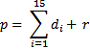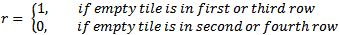14-15 Puzzle - Generation & Automatic SolvingDownload Applet with Source (116 kb)Applet Instructions

This applet allows you to investigate the so-called 14-15 puzzle - of course you can also play it :). Although I think handling of this applet is quite intuitive, here are some notes to the controls:

Movement: You can move a tile by clicking on a tile that is next to the hole - this will move the tile to the hole, e.g.
 5 12 7
clicking on tile 7 would result in
 5 7 12

Animation: check this to enable smooth movement of the tiles, otherwise they move in one step to the hole without animation. You can additionally adjust the animation speed. Note that this slider also influences the speed of the automatic solver playback - more concrete, it affects also the pause between each step of the solver.

Puzzle Generation
: The are two buttons for generating a new random puzzle - it doesn't matter which method you use (see ???? for details), but ensure to check the 'Generate only solvable puzzles' if you really want to solve the generated puzzle - otherwise there is a 50-50 chance that the created puzzle is not solvable at all.

Parity/Solvable
: Here you can calculate the parity of the current puzzle (see ???) which is used to determine if a puzzle is solvable or not.

Solver
: Press this button to start the automatic solver - the current puzzle is solved in front of your eyes!

## History

For a good starting point of the origin of this puzzle, check the article at Wikipedia.

## Solvability

As I have already stated, some puzzles (well, approximately 50%) of all puzzles are not solvable. In fact, the original 14-15 puzzle, that is the puzzle where only tiles 14 and 15 are swapped (the one you see when you start the applet) is also not solvable.
Here I present some notes along with a pseudocode algorithm that should gives you an idea why & how it works, but no mathematical prove - therefore you have to search somewhere else :-)

At first, we introduce a disorder parameter that is defined as follows: For each tile, check how many tiles before this tile have a greater value than the tile itself (reading each row from left to right and top to bottom). An example will make it clear - look at following puzzle:

 3 2 1 4 6 5 7 8 9 10 11 12 13 14 15

set disorder = 0;
Start with 3: It's the first tile so we have larger tile before it.
2: 3 is larger than 2 so increase disorder by 1.
1: 3 and 2 are larger than 1, so increase disorder by 2.
4: all tiles before are smaller than 4 - disorder remains unchanged.
6: all tiles before are smaller than 6 - disorder remains unchanged.
5: 6 is bigger than 5, all other before are smaller - increase disorder by 1
All remaining tiles are in the correct order, so they do not affect the disorder parameter. In conclusion we have disorder = 4.

For our solvability argument, we do one additional step: We add the number of the row (starting with 0, that is 0..3) to the disorder parameter. To understand this, start with the solved puzzle:

 1 2 3 4 5 6 7 8 9 10 11 12 13 14 15

It's easy to see that the disorder parameter is zero for the solved. Also note that moving the empty tile horizontally (left or right) does never change the disorder parameter.

 1 2 3 4 5 6 7 8 9 10 11 13 14 15 12

Playing around, e.g. moving the empty tile upwards, the disorder parameter changes to 3.

 1 2 3 4 5 6 7 9 10 11 8 13 14 15 12

Playing around, e.g. moving the empty tile upwards, the disorder parameter changes to 6.

If you keep playing, you will notice that the disorder parameter is even if the empty tile is in the first or third row, and it its odd when the empty tile is in the second or fourth row. Contrary to this, consider the original puzzle state:

 1 2 3 4 5 6 7 8 9 10 11 12 13 15 14

 1 2 3 4 5 6 7 8 9 10 11 13 15 14 12

If you play keep playing you'll notice that it's the other way round in this case! The disorder parameter is even if the empty tile is in the second or fourth row; it's odd if it's in the first or third row.

Using these facts, we can summarize a solvability argument by defining the parity p:where di= # tiles that are bigger than tile i and come before tile i andThe puzzle is solvable if p is even. In Pseudocode (assuming Puzzle is in array from 0 to 15 holding the puzzle tile where Value to Value are the tiles in the first row, Value to Value in the second row etc. Value[i] = 0 means the empty tile):

 BOOL isSolvable() {   int parity = 0;   for (int tile = 0; tile < 15; tile++)   {     for (int tileBefore = 0; tileBefore < tile; tileBefore++)     {       if (Puzzle[tileBefore] > Value[tile])         parity++;     }   }   // add 1 to parity if empty tile is in row 0 or 2 (and not in 1 or 3)   if ((row & 1) == 0)     parity++;   // if parity is even -> puzzle sovable   return ((parity & 1) == 0); }

## Automatic Solver

Although this is the most interesting part, I won't describe the automatic solver. In fact, the algorithm I implemented is already described - see  and . Please also note the good explanation of the special cases in  - I must admit that I started implementing the algorithm but got stuck in such these special cases, so I 'borrowed' the good solution.

Here just an overview of the performance of the solver on my 2 PCs - 2 million puzzles were generated:

Core2 T5500, 1,66Ghz, 2GB, WinXP X32
Core i5-750, Win7 X64
 Total Time (in ms) Puzzles/s
 Total Time (in ms) Puzzles/s
 135938 14712
 59492 33618

## Puzzle Generation

I did not find useful information about generating a puzzle, so I created and implemented two different algortihms; let's call them Randomize-Bruteforce-Algorithm and Randomize-Shuffling-Algorithm:

a) Randomize-Bruteforce-Algorithm:

This algorithm fills the tiles of the puzzle in random order. More precisely, again let Puzzle be an array holding the sixteen tiles, for each row from top to bottom. At first we fill the array in increasing order, thus Puzzle = 0, Puzzle = 1 ... so we put each tile into the puzzle array. Then we loop over the array and swap randomly two tiles. After this step we have a completely randomized puzzle - but here lies the first disadvantage: The puzzle we get may be solvable or not. If we want explicitely a solvable puzzle, then we have to check the puzzle for solvability after each randomization and repeat the procedure if necessary. This results in the second trap: In theory this algorithm could run forever if each time an unsolvable puzzle is generated. The probability that the puzzle is unsolvable is approximately 1/2 = 50% for one iteration of this algorithm and (1/2)n after n iterations. So e.g. after n = 10 iterations, the probability that still no solvable puzzle is generated is (1/2)10 < 0,1% but still possible. Therefore, a maximum number of iterations should be defined after the algorithm aborts in every case. In Pseudocode:

 void Randomize_Bruteforce() {   const int NumTries = 50;   // first fill the puzzle in increasing order   for (int i = 0; i < 16; i++)     Puzzle[i] = i;      int rnd, swap;   int tries = 0;   while (!isSolvable() && tries <= NumTries)   {     // generate random array by swapping two tiles     for (int k = 0; k < 16; k++)     {       // calculate new random position for tile at position k       rnd = (int)((15-k+1) * Math.random() + k);       // swap the tiles at positions k and rnd       swap = Puzzle[k];       Puzzle[k] = Puzzle[rnd];       Puzzle[rnd] = swap;     }     tries++;   } }

b) Randomize-Shuffling-Algorithm:

This algorithm follows another idea. Here we start with the solved puzzle. Then we move the empty space by one position where the direction of the movement is randomly. We repeat this step for a predefined number of movements or until we think the puzzle is 'randomly enough' - I chose the first termination condition as I was to lazy to think about a good metric to classify a puzzle as random or not. This has also the advantage that the algorithm always terminates with a quite balanced execution time. Moreover, the algorithm always produces a solvable puzzle. The drawback of this approach was releaved while testing - for a good 'random' puzzle I suggest at least 100 movements of the empty tile which makes the algorithm slower in average compared to my bruteforce apporach. Here the pseudocode:

 void Randomize_Shuffling() {   const int NUM_OF_STEPS = 100;   InitPuzzleArrayWithTheSolvedPuzzle();   int steps = 0;   while (steps < NUM_OF_STEPS)   {     rnd = (int)(Math.random() * 4);    /* generated random value of 0,1,2 or 3 */     switch (rnd)     {       case 0: MoveEmptyTileUpwardsIfPossible(); break;       case 1: MoveEmptyTileDownwardsIfPossible(); break;       case 2: MoveEmptyTileToTheLeftIfPossible(); break;       case 3: MoveEmptyTileToTheRightIfPossible(); break;       default: break;    /* should not happen */     }     steps++;   } }

c) Performance Overview:

Just a short overview of both algorithms on my PC (in average)- again 2 million puzzles were generated:

Core2 T5500, 1,66Ghz, 2GB, WinXP X32
Core i5-750, Win7 X64

 Total Time (in ms) Puzzles/s
 Total Time (in ms) Puzzles/s
Randomize-Shuffling-Algorithm
(NUM_OF_STEPS = 50)
 14750 135593
 3500 572409
Randomize-Shuffling-Algorithm
(NUM_OF_STEPS = 100)
 28969 69039
 6864 291375
Randomize-Bruteforce-Algorithm
 13438 148831
 4648 430292

References:

Sunshine, February 2010

This site is part of Sunshine's Homepage